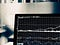Noob v. LeetCode, Episode 8, array manipulationPhoto by Chris Liverani on Unsplash

Looking through LeetCode’s top interview questions in the ‘easy’ category, I found this one:

My first approach went like this. For each number in the array- let’s call it buy — find the greatest element to the right — let’s call that one sell. If sell is lower than buy, let’s subtract sell from buy and call the result profit.

Meanwhile, we’ll have another variable called maxProfit that starts at zero. At the end of each loop, if profit is greater than maxProfit, then we’ll set maxProfit equal to profit. If that confuses you, I’ll break it down into steps below.

Step 1. Iterate, while keeping track of index

var maxProfit = function(prices) {
let profit
let maxProfit = 0

// more code here
})
return maxProfit
};

Step 2. Make a new sub-array of elements to the right

let rest = prices.slice(index + 1)
//it's index + 1 because we want all elements to the right ...

Step 3. Find the biggest number in that sub-array

But … if we’re looking at the last element of the array, then rest = []. To account for this edge case, let’s first check to see that rest is not null. We’ll use javaScript’s Math.max() to find the largest values. We’ll save that value as sell.

if(rest){
let sell = Math.max(...rest)
}

Step 4. Calculate profit

If sell is greater than buy (as required by rules of this challenge), we can calculate profit like so:

if (sell > buy){
profit = sell — buy
}

Step 5. Check to see if profit is greater than maxProfit

For each buy, we are calculating profit. We started maxProfit at zero. Now, inside our loop, we need to compare the two, and always assign the greater value to maxProfit:

profit > maxProfit ? maxProfit = profit : null

Finally, at the end of our loop, we return maxProfit:

var maxProfit = function(prices) {
let profit
let maxProfit = 0

let rest = prices.slice(index + 1)
if (rest){
let sell = Math.max(...rest)
sell > buy ? profit = sell - buy : null
profit > maxProfit ? maxProfit = profit : null
}
})
return maxProfit
};

It works! BUT how does it perform?

We are in the bottom 5 percent!

Why? Because we have nested loops — an if loop inside a for loop, not to mention two ternary operations for each nested loop.

We need a different approach!

Our first approach was to break our array into two arrays, nesting one inside the other. This time, let’s iterate only once. We’ll still set an initial value for maxProfit. We’ll also set an initial value for min (minimum value, ie lowest price).

var maxProfit = function(prices) {
let maxProfit = 0;
let min = prices;
for(let i = 1; i < prices.length; i++) {
// more code here
}
}

As we iterate we’ll compare our most recent value for min with the next element, and set the lesser of those two values as the new value for min. We’re using javaScript’s Math.min().

const prices = [7,1,5,3,6,4]
min = Math.min(prices[i], min);
//i = 1, min = Math.min(1,7) => 1

Tracking Profits

In the same loop, we also update maximum profit, which we define as either the previous value for maxProfit, or the current price minus min.

maxProfit = Math.max(maxProfit, prices[i] - min);

Here’s a look at how those values update after each loop:

const prices = [7,1,5,3,6,4]for(let i = 1; i < prices.length; i++) {        min = Math.min(prices[i], min);
//i = 1, lowestPrice = Math.min(1,7) => 1
//i = 2, lowestPrice = Math.min(5,1) => 1
//i = 3, lowestPrice = Math.min(3,1) => 1
//i = 4, lowestPrice = Math.min(6,1) => 1
//i = 5, lowestPrice = Math.min(4,1) => 1
maxProfit = Math.max(maxProfit, prices[i] - min);
//i = 1, Math.max(0, 1 - 1) => 0
//i = 2, Math.max(0, 5 - 1) => 4
//i = 3, Math.max(4, 3 - 1) => 4
//i = 4, Math.max(4, 6 - 1) => 5
//i = 5, Math.max(5, 4 - 1) => 5
}

All together now:

const maxProfit = function(prices) {
let maxProfit = 0;
let lowestPrice = prices;
for(let i = 1; i < prices.length; i++) {
min = Math.min(prices[i], min);
maxProfit = Math.max(maxProfit, prices[i] - min);
}
return maxProfit;
};

It works! And this time we are only using one loop, plus Math.max() and Math.min():

next: Algorithms 101, #9: Jewels and Stones in Ruby and JS

in case you missed it: Algorithms 101, #7: House Robber in JavaScript

Written by

JavaScript in Plain English

Learn the web's most important programming language.

Welcome to a place where words matter. On Medium, smart voices and original ideas take center stage - with no ads in sight. Watch
Follow all the topics you care about, and we’ll deliver the best stories for you to your homepage and inbox. Explore
Get unlimited access to the best stories on Medium — and support writers while you’re at it. Just \$5/month. Upgrade